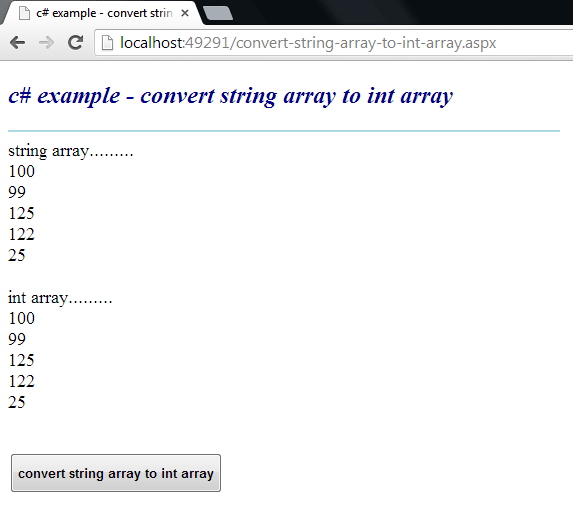### c# - How to convert a string array to an int array

Convert a String Array to an Int Array
The Array class provides methods for creating, manipulating, searching, and sorting arrays. The Array class is not part of the System.Collections namespaces. However, it is still considered a collection because it is based on the IList interface. An element is a value in an Array. The length of an Array is the total number of elements it can contain. The Array has a fixed capacity.

The following .net c# tutorial code demonstrates how we can convert a String Array to an Int Array. That means we will convert a String Array instance to an Int Array instance where all the elements of the Srting Array are the String representations of int values. But there is no direct method to convert a String Array object to an Int Array instance. So, we have to perform some jobs to get an Int Array from String Array elements.

At first, we have to initialize an empty instance of an Int Array. While initializing the Int Array instance, we will set the Int Array size exactly the same as the String Array size. After that, we will loop through the String Array elements. While iterating the String Array elements, we will set the Int Array’s elements value corresponding to the String Array elements.

While setting the Int Array elements value we have to convert the String value to Int instance. In this .net example code, we will use the Convert class ToInt32() method to convert a String instance to an integer instance. So finally, we will get an Int Array instance from the String Array elements.

The Convert class converts a base data type to another base data type. The Convert class ToInt32() method converts a specified value to a 32-bit signed integer.

The Convert class ToInt32(String) method overload converts the specified string representation of a number to an equivalent 32-bit signed integer. The Convert class ToInt32 (string? value) method overload has a parameter named value.

The value parameter is a string that contains the number to convert. The Convert class ToInt32(String) method returns a 32-bit signed integer that is equivalent to the number in value, or 0 if the value is null.

The Convert class ToInt32(string? value) method overload throws FormatException if the value does not consist of an optional sign followed by a sequence of digits (0 through 9). It also throws OverflowException if the value represents a number that is less than Int32.MinValue or greater than Int32.MaxValue.
convert-string-array-to-int-array.aspx
``````
<%@ Page Language="C#" AutoEventWireup="true"%>

<!DOCTYPE html>
<script runat="server">
protected void Button1_Click(object sender, System.EventArgs e)
{
string[] stringarray = new string[]
{
"100",
"99",
"125",
"122",
"25"
};

Label1.Text = "string array.........<br />";
foreach (string s in stringarray)
{
Label1.Text += s + "<br />";
}

int[] intarray = new int[stringarray.Length];
for (int i = 0; i < stringarray.Length;i++ )
{
intarray[i] = Convert.ToInt32(stringarray[i]);
}

Label1.Text += "<br />int array.........<br />";
foreach (double d in intarray)
{
Label1.Text += d.ToString() + "<br />";
}
}
</script>

<html xmlns="http://www.w3.org/1999/xhtml">
<title>c# example - convert string array to int array</title>
<body>
<form id="form1" runat="server">
<div>
<h2 style="color:DarkBlue; font-style:italic;">
c# example - convert string array to int array
</h2>
<hr width="550" align="left" color="LightBlue" />

<asp:Label
ID="Label1"
runat="server"
Font-Size="Large"
>
</asp:Label>
<br /><br />
<asp:Button
ID="Button1"
runat="server"
Text="convert string array to int array"
OnClick="Button1_Click"
Height="40"
Font-Bold="true"
/>
</div>
</form>
</body>
</html>
``````More c# examples